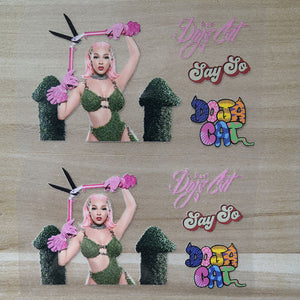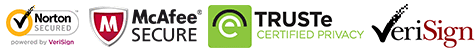FedEx will stop during Oct 1-8
FedEx will stop during Oct 1-8# Doja Cat Iron On Patches For Custom Air Force 1, Heat Transfer Sticker for Custom Shoes Doja Cat

Regular price \$15.00 \$9.90 Save \$5.10

ONLY 199856 LEFT

 Hours   Minutes   Seconds

Sale Ends Once The Timer Hits Zero!

ONLY 199856 LEFT

Get the easy use Doja Cat iron on patches to custom your own Doja Cat AF1

or Vans. Easy use to DIY and make perfect gift for her.

<<<<<<<<<<<<<<<<<<<<<<<<<<<<<<<<<<<<<<<<<<<<<<<<<<

Why use these Doja Cat patches:

1. Perfect sizes for sneakers: AF1, Vans, Converse or other shoes.

2. Also can be used on cloth, socks. hoodies, jeans, tshirt....

3. Durable, waterproof and flexible.

4. Easy use, just need to iron on top.

5. Get these Doja Cat patches to DIY, save lot's money to buy Doja Cat shoes.

5. They are durable while still can be removed, take 1-2 hours and patient,
they can removed piece by piece. When you tired this design,
can change to iron another design, to refresh your shoes.

<<<<<<<<<<<<<<<<<<<<<<<<<<<<<<<<<<<<<<<<<<<<<<<<<

The set includes
2 x Doja Cat
4 x Doja Cat names
2 x say so

<<<<<<<<<<<<<<<<<<<<<<<<<<<<<<<<<<<<<<<<<<<<<<<<<<

How to use:

1. Position the Doja Cat and other small designs on the shoes with tape

2. Iron on the top of the Doja Cat, please keep temperature 130-150℃,
and iron slightly

3. peel the vinyl, the Doja Cat patches will stick on the shoes.

4. If use normal iron, please turn off the steam, keep the temperature above,
use the tip and be careful and patient, for it's heavy to control, better use the
mini iron.

5. You can watch the tutorial video here:

the process is the same.

<<<<<<<<<<<<<<<<<<<<<<<<<<<<<<<<<<<<<<<<<<<<<<<<<<<

Free shipping

people are currently looking at this product

Estimated delivery time 7-15 days

Guaranteed Safe Checkout